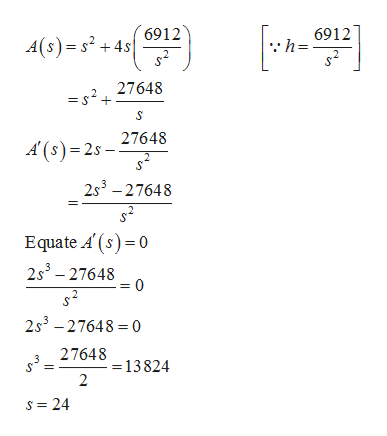# A rectangular tank with a square base, an open top, and a volume of 6912 ft^2 is to be constructed of sheet steel. Find the dimensions of the tank that has the minimum surface area.Let s be the length of one of the sides of the square base and let A be the surface area of the tank. Write the objective function.A= _____ (type an expression)The tank with the minimum surface area has a height of ___ ft and a square base with a sidelength of ___ ft.

Question
198 views

A rectangular tank with a square base, an open top, and a volume of 6912 ft^2 is to be constructed of sheet steel. Find the dimensions of the tank that has the minimum surface area.

Let s be the length of one of the sides of the square base and let A be the surface area of the tank. Write the objective function.

A= _____ (type an expression)

The tank with the minimum surface area has a height of ___ ft and a square base with a sidelength of ___ ft.

check_circle

Step 1

Given tank is open at top.

So, the surface area is,

Step 2

Find the minimum surface...help_outlineImage Transcriptionclose6912 6912 A(s) s2+4s h= 27648 27648 A(s) 2s- 2s3-27648 Equate A' (s) 0 2s3-27648 2s3-27648 0 27648 13824 s 24 fullscreen

### Want to see the full answer?

See Solution

#### Want to see this answer and more?

Solutions are written by subject experts who are available 24/7. Questions are typically answered within 1 hour.*

See Solution
*Response times may vary by subject and question.
Tagged in

### Other